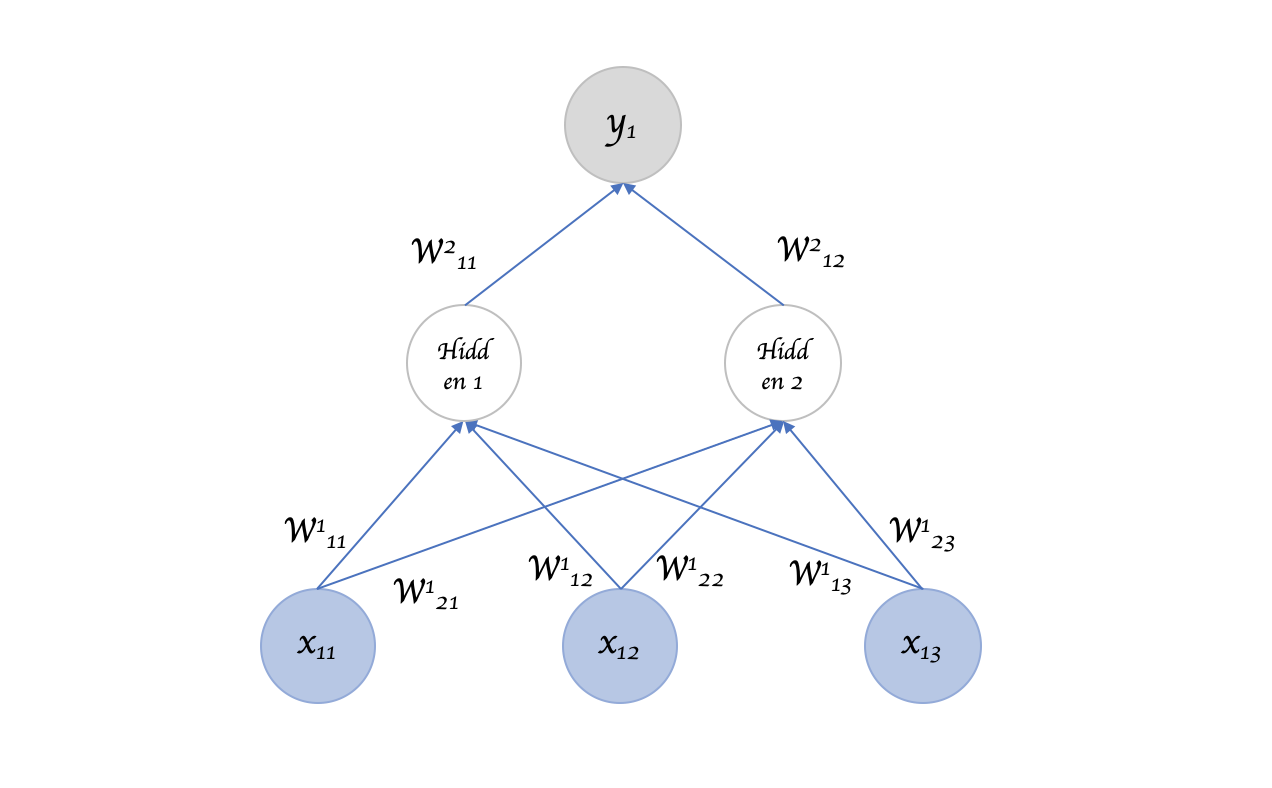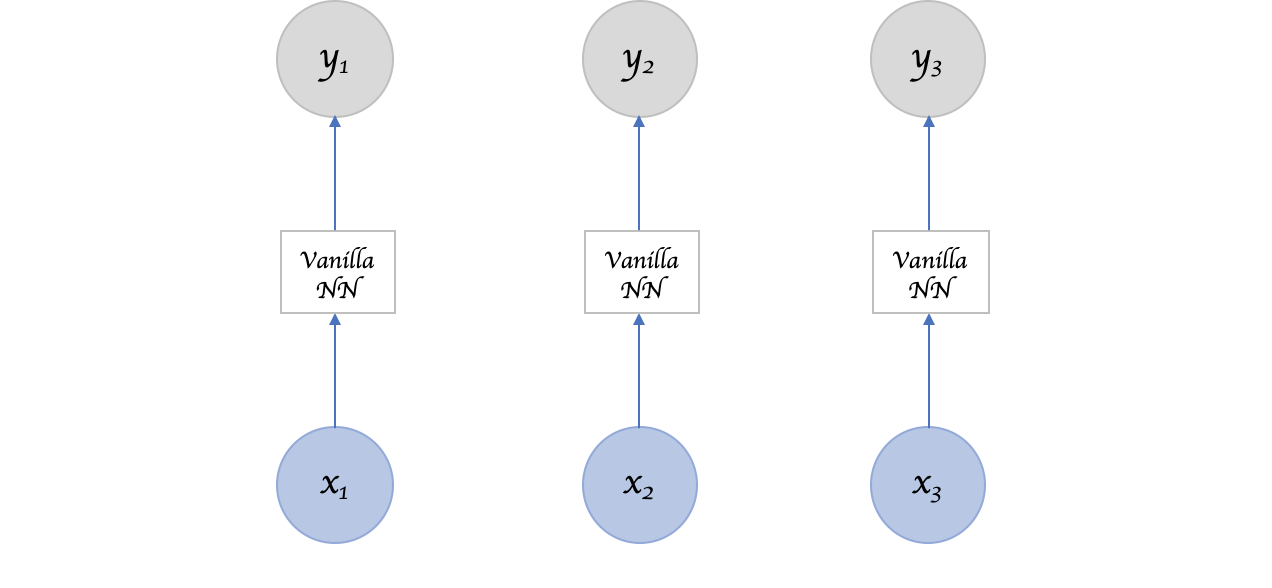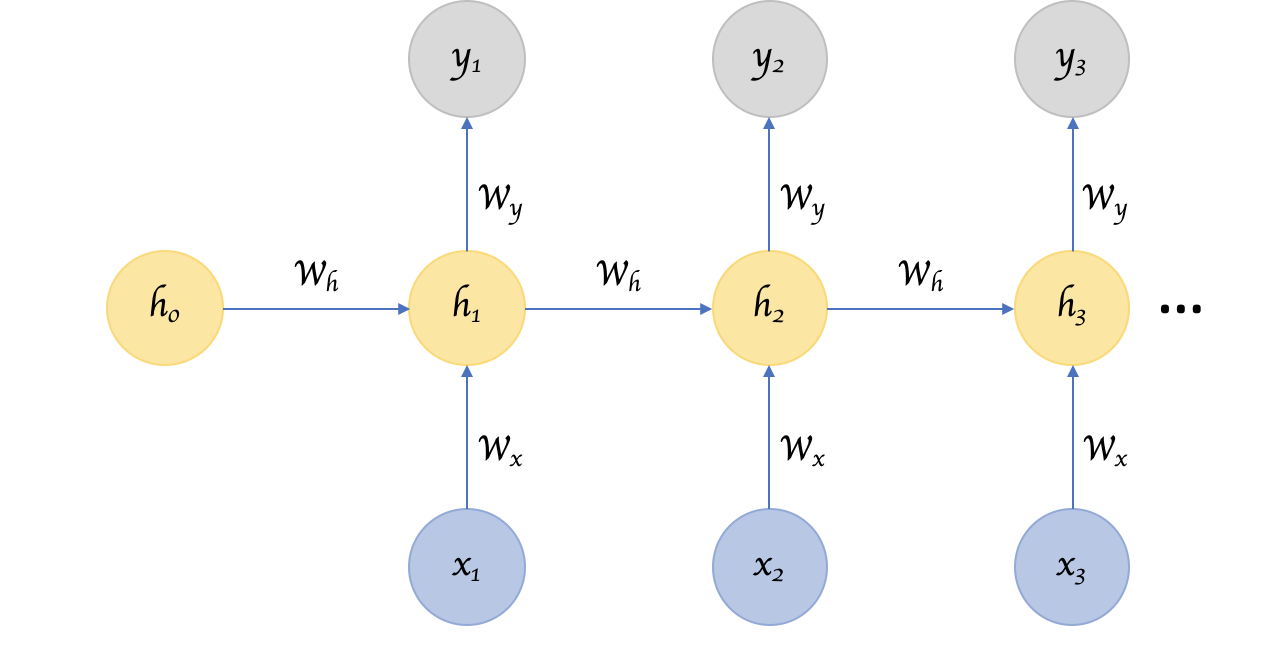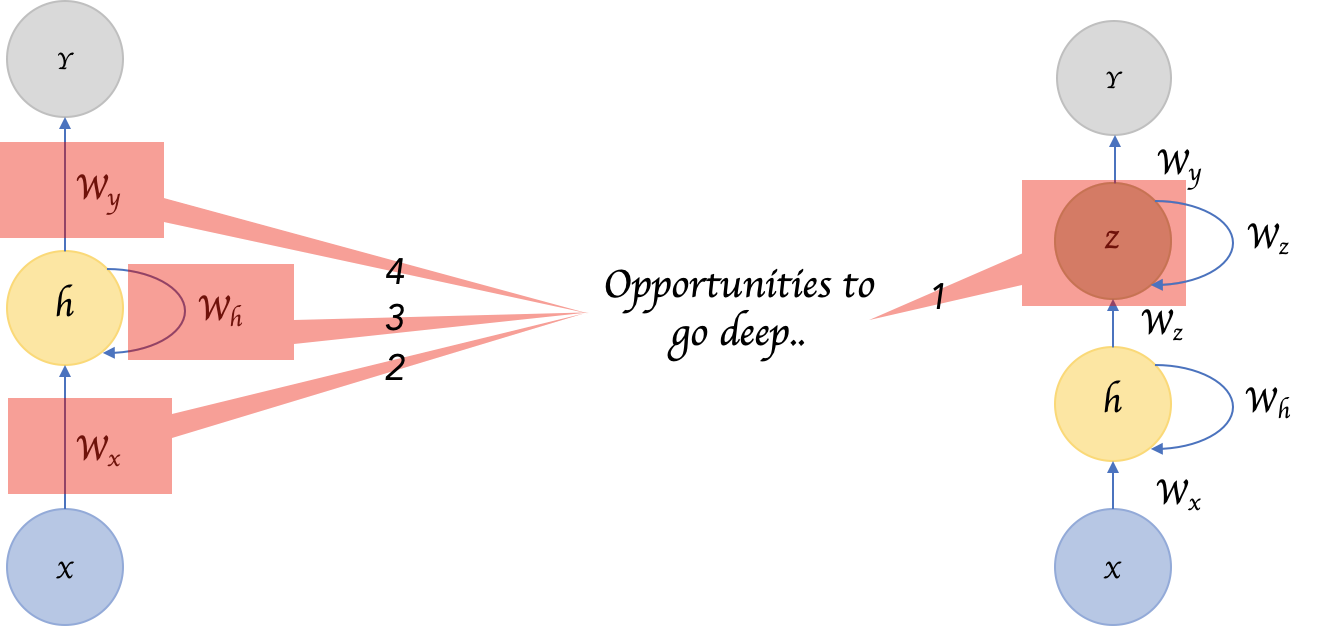# Recurrent Neural Networks – Remembering what’s important

Recurrent Neural Networks (RNNs) add an interesting twist to basic neural networks. A vanilla neural network takes in a fixed size vector as input which limits its usage in situations that involve a ‘series’ type input with no predetermined size. Whereas RNNs are designed to take a series of input with no predetermined limit on size.

Recurrent Neural Networks (RNNs) add an interesting twist to basic neural networks. A vanilla neural network takes in a fixed size vector as input which limits its usage in situations that involve a ‘series’ type input with no predetermined size.Figure 1: A vanilla network representation, with an input of size 3 and one hidden layer and one output layer of size 1.

RNNs are designed to take a series of input with no predetermined limit on size. One could ask what’s the big deal, I can call a regular NN repeatedly too?Figure 2: Can I simply not call a vanilla network repeatedly for a ‘series’ input?

Sure can, but the ‘series’ part of the input means something. A single input item from the series is related to others and likely has an influence on its neighbors. Otherwise it’s just “many” inputs, not a “series” input (duh!).

So we need something that captures this relationship across inputs meaningfully.

## Recurrent Neural Networks

Recurrent Neural Network remembers the past and it’s decisions are influenced by what it has learnt from the past. Note: Basic feed forward networks “remember” things too, but they remember things they learnt during training. For example, an image classifier learns what a “1” looks like during training and then uses that knowledge to classify things in production.

While RNNs learn similarly while training, in addition, they remember things learnt from prior input(s) while generating output(s). It’s part of the network. RNNs can take one or more input vectors and produce one or more output vectors and the output(s) are influenced not just by weights applied on inputs like a regular NN, but also by a “hidden” state vector representing the context based on prior input(s)/output(s). So, the same input could produce a different output depending on previous inputs in the series.Figure 3: A Recurrent Neural Network, with a hidden state that is meant to carry pertinent information from one input item in the series to others.

In summary, in a vanilla neural network, a fixed size input vector is transformed into a fixed size output vector. Such a network becomes “recurrent” when you repeatedly apply the transformations to a series of given input and produce a series of output vectors. There is no pre-set limitation to the size of the vector. And, in addition to generating the output which is a function of the input and hidden state, we update the hidden sate itself based on the input and use it in processing the next input.

## Parameter Sharing

You might have noticed another key difference between Figure 1 and Figure 3. In the earlier, multiple different weights are applied to the different parts of an input item generating a hidden layer neuron, which in turn is transformed using further weights to produce an output. There seems to be a lot of weights in play here. Whereas in Figure 3, we seem to be applying the same weights over and over again to different items in the input series.

I am sure you are quick to point out that we are kinda comparing apples and oranges here. The first figure deals with “a” single input whereas the second figure represents multiple inputs from a series. But nevertheless, intuitively speaking, as the number of inputs increase, shouldn’t the number of weights in play increase as well? Are we losing some versatility and depth in Figure 3?

Perhaps we are. We are sharing parameters across inputs in Figure 3. If we don’t share parameters across inputs, then it becomes like a vanilla neural network where each input node requires weights of their own. This introduces the constraint that the length of the input has to be fixed and that makes it impossible to leverage a series type input where the lengths differ and is not always known.

But what we seemingly lose in value here, we gain back by introducing the “hidden state” that links one input to the next. The hidden state captures the relationship that neighbors might have with each other in a serial input and it keeps changing in every step, and thus effectively every input undergoes a different transition!

Image classifying CNNs have become so successful because the 2D convolutions are an effective form of parameter sharing where each convolutional filter basically extracts the presence or absence of a feature in an image which is a function of not just one pixel but also of its surrounding neighbor pixels.

In other words, the success of CNNs and RNNs can be attributed to the concept of “parameter sharing” which is fundamentally an effective way of leveraging the relationship between one input item and its surrounding neighbors in a more intrinsic fashion compared to a vanilla neural network.

## Deep RNNs

While it’s good that the introduction of hidden state enabled us to effectively identify the relationship between the inputs, is there a way we can make a RNN “deep” and gain the multi level abstractions and representations we gain through “depth” in a typical neural network?Figure 4: We can increase depth in three possible places in a typical RNN. This paper by Pascanu et al., explores this in detail.

Here are four possible ways to add depth. (1) Perhaps the most obvious of all, is to add hidden states, one on top of another, feeding the output of one to the next. (2) We can also add additional nonlinear hidden layers between input to hidden state (3) We can increase depth in the hidden to hidden transition (4) We can increase depth in the hidden to output transition. This paper by Pascanu et al., explores this in detail and in general established that deep RNNs perform better than shallow RNNs.

## Bidirectional RNNs

Sometimes it’s not just about learning from the past to predict the future, but we also need to look into the future to fix the past. In speech recognition and handwriting recognition tasks, where there could be considerable ambiguity given just one part of the input, we often need to know what’s coming next to better understand the context and detect the present.

This does introduce the obvious challenge of how much into the future we need to look into, because if we have to wait to see all inputs then the entire operation will become costly. And in cases like speech recognition, waiting till an entire sentence is spoken might make for a less compelling use case. Whereas for NLP tasks, where the inputs tend to be available, we can likely consider entire sentences all at once. Also, depending on the application, if the sensitivity to immediate and closer neighbors is higher than inputs that come further away, a variant that looks only into a limited future/past can be modeled.

## Recursive Neural Networks

A recurrent neural network parses the inputs in a sequential fashion. A recursive neural network is similar to the extent that the transitions are repeatedly applied to inputs, but not necessarily in a sequential fashion. Recursive Neural Networks are a more general form of Recurrent Neural Networks. It can operate on any hierarchical tree structure. Parsing through input nodes, combining child nodes into parent nodes and combining them with other child/parent nodes to create a tree like structure. Recurrent Neural Networks do the same, but the structure there is strictly linear. i.e. weights are applied on the first input node, then the second, third and so on.

But this raises questions pertaining to the structure. How do we decide that? If the structure is fixed like in Recurrent Neural Networks then the process of training, backprop etc makes sense in that they are similar to a regular neural network. But if the structure isn’t fixed, is that learnt as well? This paper and this paper by Socher et al., explores some of the ways to parse and define the structure, but given the complexity involved, both computationally and even more importantly, in getting the requisite training data, recursive neural networks seem to be lagging in popularity to their recurrent cousin.

## Encoder Decoder Sequence to Sequence RNNs

Encoder Decoder or Sequence to Sequence RNNs are used a lot in translation services. The basic idea is that there are two RNNs, one an encoder that keeps updating its hidden state and produces a final single “Context” output. This is then fed to the decoder, which translates this context to a sequence of outputs. Another key difference in this arrangement is that the length of the input sequence and the length of the output sequence need not necessarily be the same.